# Autocorrelation functions of individual charge dipole moments in DL_MESO_DPD¶

## Purpose of Module¶

This module, gen_moldipaf.f90, is a post-processing utility for DL_MESO_DPD, the Dissipative Particle Dynamics (DPD) code from the DL_MESO package.

It processes the trajectory (HISTORY) files to obtain the charge dipole moments of all the (neutral) molecules in the system, and subsequently computes the dipole autocorrelation functions (DAFs) for individual molecules, for each molecule type. It produces a file MDIPAFDAT containing both the un-normalized and normalized DAFs, and, optionally, a file MDIPAFFFT containing the Fourier transform (FT) of the latter. It is analogous to gen_dipoleaf.f90, but deals with individual (for a single molecule) rather then macroscopic (for the simulated volume) charge dipole moments.

The module can be applied to systems including molecules with a generic charge structure, as long as each molecule is neutral (otherwise the charge dipole moment would be frame-dependent) . CAVEAT: this module only analyzes molecular trajectories. If a charged molecule is present, an error message will be given, while unbonded charges would not be detected and would lead to erroneous results. Therefore please keep in mind to not apply the module to systems with unbonded charges.

The charge dipole moment of a neutral molecule is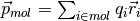where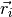are the bead positions and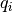their charges. The total charge dipole moment of the simulated volume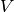is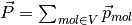. If more than one molecular species are present, one can split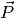into the different species contributions:, where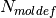is the number of molecule types (definitions) and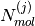the number of molecules of type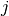.

Given a scalar quantity A, its non-normalized autocorrelation function (AF) is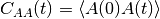, where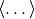indicates an average over trajectories. The normalized one is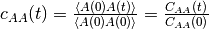.

Here for the-th molecular species we replace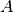with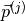, and the product with a scalar product. In this case the average over trajectories translates into two sums, one over different time origins and one over molecules of species.

The output file MDIPAFDAT contains the DAFs for each molecular species and, at the end of the file, the DAF obtained averaging over all the particles. The output file MDIPAFFFT contains the FT of these functions, in the same order.

More in detail, the header of the file MDIPAFDAT contains the simulation title and a line with the number of snapshots in HISTORY and of those used for the AFs (naf). Then a block follows for each molecule type, started by the {molecule name}, then three columns of data,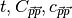. It is intended that in any block only the molecules for a given species are summed over. The last block is called {all species} and refers to an average over all the molecules.

The header of the file MDIPAFFFT is as for MDIPAFDAT (notice that the number of points for the FT is also set equal to naf). Then a block follows for each molecule type, started by the molecule name, then three columns of data,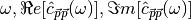, where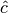is the discrete FT of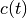.

Possible uses of the output file are: to analyze it to obtain the decay time of autocorrelations, which can be used to define an efficient sampling of the simulation; to compare it with the analogous macroscopic one obtained for all the molecules (of a given type) in the system (see Autocorrelation functions of charge dipole moments in DL_MESO_DPD).

## Background Information¶

The base code for this module is DL_MESO_DPD, the Dissipative Particle Dynamics code from the mesoscopic simulation package DL_MESO, developed by M. Seaton at Daresbury Laboratory. This open source code is available from STFC under both academic (free) and commercial (paid) licenses. The module is to be used with DL_MESO in its last released version, version 2.6 (dating November 2015).

Also, the present module requires the library FFTW (version 3.x) to be installed.

## Testing¶

The present module gen_moldipaf.f90 is compiled with the available Fortran90 compiler, e.g.:

gfortran -lfftw3 -lm -o gen_moldipaf.exe gen_moldipaf.f90

and the executable must be in the same directory of the HISTORY* files to be analyzed. In case the file fftw3.f, containing constants that are necessary for the Fourier transform, is not found by the compiler, a simple way out is to copy it in the same directory where the module is run. The user is asked to provide the number of nodes used to run the simulation, the electric charges for all the types of beads and the maximum number of snapshots to be used for the AFs (naf). Finally, the last input is a switch for the Fourier transform: 1 for yes, 0 (or any other integer) for no.

To input these parameters one can enter them from the keyboard or write them into a text file (say, input.txt), one per line (in the right order) and run the program in this way:

gen_dipoleaf.exe < input.txt

Test: water in oil

As a test, we suggest to consider a fluid made of harmonically bonded dimers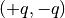. Fixing appropriately the partial charge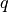and the Bjerrum length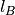this system mimics water in an oil background, as long as the dielectric properties are concerned. For more details about this model, please see the page Test case: a dimer solvent.

Run DL_MESO_DPD using for the CONTROL file

DL_MESO charged harmonic dimers with dpd repulsion

volume 64.0
temperature 1.0
cutoff 1.0

timestep 0.01
steps 70000
equilibration steps 20000
traj 20000 10
stats every 100
stack size 100
print every 100
job time 7200.0
close time 10.0

ensemble nvt mdvv

ewald sum 1.0 5 5 5
bjerrum 42.0
smear gauss equal

finish


and for the FIELD file

DL_MESO charged harmonic dimers with dpd repulsion

SPECIES 2
solp  1.0   0.46  0
solm  1.0  -0.46  0

MOLECULES 1
DIMER
nummols 96
solp  0.0 0.0 0.0
solm  0.1 0.0 0.0
bonds 1
harm 1 2 5.0 0.0

finish

INTERACTIONS 3
solp  solp  dpd 25.0 1.0 4.5
solm  solm  dpd 25.0 1.0 4.5
solp  solm  dpd 25.0 1.0 4.5

CLOSE


Analyzing the HISTORY file with gen_moldipaf.exe choosing naf=100, i.e., using this input.txt (which assumes a serial run)

1
0.46
-0.46
100
1


this output is printed on the standard output

 Number of nodes used in calculations ?
nchist:            0          96           0           0           0           0           0           0           0           0
Charges for SPECIES type solp     :
Charges for SPECIES type solm     :
chg=       0.4600      -0.4600
Number of time steps in autocorrelation profile?
switch for FFT computation? (1=yes, 0 or any other integer=no)


The first line shows the histogram of cluster sizes: in this case, it correctly gives 96 molecules of two beads. Since internally the module checks that each molecule is a connected cluster , this line should always give a histogram with the molecule sizes (by default, shown up to ten beads).

The MDIPAFDAT file is (only the first fifteen lines are shown)

DL_MESO charged harmonic dimers with dpd repulsion
5001       100

DIMER
0.000000E+00  1.419184E-01  1.000000E+00
1.000000E-01  1.360256E-01  9.584780E-01
2.000000E-01  1.217816E-01  8.581103E-01
3.000000E-01  1.045570E-01  7.367406E-01
4.000000E-01  8.750295E-02  6.165724E-01
5.000000E-01  7.161709E-02  5.046358E-01
6.000000E-01  5.723649E-02  4.033057E-01
7.000000E-01  4.466587E-02  3.147293E-01
8.000000E-01  3.406310E-02  2.400189E-01
9.000000E-01  2.537519E-02  1.788013E-01


and the MDIPAFFFT file is (only the first fifteen lines are shown)

DL_MESO charged harmonic dimers with dpd repulsion
5001       100

DIMER
0.000000E+00  6.013063E+00  0.000000E+00
6.283185E-01  5.915405E+00 -1.378369E+00
1.256637E+00  5.283007E+00 -2.365590E+00
1.884956E+00  4.077657E+00 -3.150028E+00
2.513274E+00  3.076368E+00 -3.326590E+00
3.141593E+00  2.244706E+00 -3.195932E+00
3.769911E+00  1.631808E+00 -2.873471E+00
4.398230E+00  1.273571E+00 -2.521504E+00
5.026548E+00  1.024723E+00 -2.219809E+00
5.654867E+00  8.805952E-01 -1.950491E+00


Below we show a plot of the normalized AF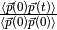(obtained using the first and third columns of MDIPAFDAT)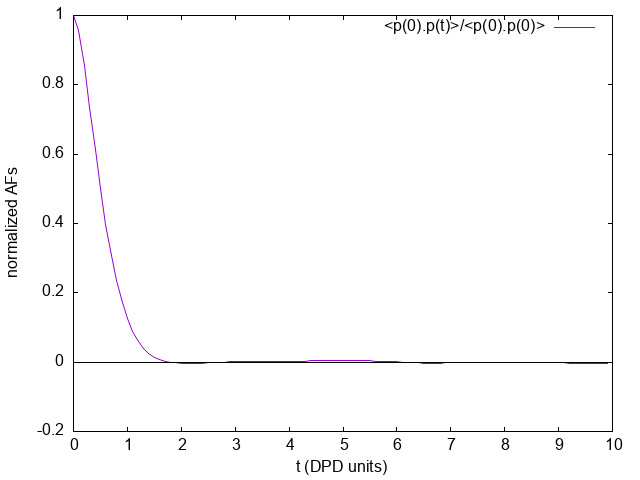## Source Code¶

  1 2 3 4 5 6 7 8 9 10 11 12 13 14 15 16 17 18 19 20 21 22 23 24 25 26 27 28 29 30 31 32 33 34 35 36 37 38 39 40 41 42 43 44 45 46 47 48 49 50 51 52 53 54 55 56 57 58 59 60 61 62 63 64 65 66 67 68 69 70 71 72 73 74 75 76 77 78 79 80 81 82 83 84 85 86 87 88 89 90 91 92 93 94 95 96 97 98 99 100 101 102 103 104 105 106 107 108 109 110 111 112 113 114 115 116 117 118 119 120 121 122 123 124 125 126 127 128 129 130 131 132 133 134 135 136 137 138 139 140 141 142 143 144 145 146 147 148 149 150 151 152 153 154 155 156 157 158 159 160 161 162 163 164 165 166 167 168 169 170 171 172 173 174 175 176 177 178 179 180 181 182 183 184 185 186 187 188 189 190 191 192 193 194 195 196 197 198 199 200 201 202 203 204 205 206 207 208 209 210 211 212 213 214 215 216 217 218 219 220 221 222 223 224 225 226 227 228 229 230 231 232 233 234 235 236 237 238 239 240 241 242 243 244 245 246 247 248 249 250 251 252 253 254 255 256 257 258 259 260 261 262 263 264 265 266 267 268 269 270 271 272 273 274 275 276 277 278 279 280 281 282 283 284 285 286 287 288 289 290 291 292 293 294 295 296 297 298 299 300 301 302 303 304 305 306 307 308 309 310 311 312 313 314 315 316 317 318 319 320 321 322 323 324 325 326 327 328 329 330 331 332 333 334 335 336 337 338 339 340 341 342 343 344 345 346 347 348 349 350 351 352 353 354 355 356 357 358 359 360 361 362 363 364 365 366 367 368 369 370 371 372 373 374 375 376 377 378 379 380 381 382 383 384 385 386 387 388 389 390 391 392 393 394 395 396 397 398 399 400 401 402 403 404 405 406 407 408 409 410 411 412 413 414 415 416 417 418 419 420 421 422 423 424 425 426 427 428 429 430 431 432 433 434 435 436 437 438 439 440 441 442 443 444 445 446 447 448 449 450 451 452 453 454 455 456 457 458 459 460 461 462 463 464 465 466 467 468 469 470 471 472 473 474 475 476 477 478 479 480 481 482 483 484 485 486 487 488 489 490 491 492 493 494 495 496 497 498 499 500 501 502 503 504 505 506 507 508 509 510 511 512 513 514 515 516 517 518 519 520 521 522 523 524 525 526 527 528 529 530 531 532 533 534 535 536 537 538 539 540 541 542 543 544 545 546 547 548 549 550 551 552 553 554 555 556 557 558 559 560 561 562 563 564 565 566 567 568 569 570 571 572 573 574 575 576 577 578 579 580 581 582 583 584 585 586 587 588 589 590 591 592 593 594 595 596 597 598 599 600 601 602 603 604 605 606 607 608 609 610 611 612 613 614 615 616 617 618 619 620 621 622 623 624 625 626 627 628 629 630 631 632 633 634 635 636 637 638 639 640 641 642 643 644 645 646 647 648 649 650 651 652 653 654 655 656 657 658 659 660 661 662 663 664 665 666 667 668 669 670 671 672 673 674 675 676 677 678 679 680 681 682 683 684 685 686 687 688 689 690 691 692 693 694 695 696 697 698 699 700 701 702 703 704 705 706 707 708 709 710 711 712 713 714 715 716 717 718 719 720 721 722 723 724 725 726 727 728 729 730 731 732 733 734 735 736 737 738 739 740 741 742 743 744 745 746 747 748 749 750 751 752 753 754 755 756 757 758 759 760 761 762 763 764 765 766 767 768 769 770 771 772 773 774 775 776 777 778 779 780 781 782 783 784 785 786 787 788 789 790 791 792 793 794 795 796 797 798 799 800 801 802 803 804 805 806 807 808 809 810 811 812 813 814 815 816 817 818 819 820 821 822 823 824 825 826 827 828 829 830 831 832 833 834 835 836 837 838 839 840 841 842 843 844 845 846 847 848 849 850 851 852 853 854 855 856 857 858 859 860 861 862 863 864 865 866 867 868 869 870 871 872 873 874 875 876 877 878 879 880 881 882 883 884 885 886 887 888 889 890 891 892 PROGRAM gen_moldipaf !************************************************************************************* ! module to compute autocorrelation functions of individual charge dipole moments in ! DL_MESO_DPD ! ! authors: m. a. seaton and s. chiacchiera, March 2017 (amended August 2017) !************************************************************************************* IMPLICIT none INTEGER, PARAMETER :: dp = SELECTED_REAL_KIND (15, 307) INTEGER, PARAMETER :: ntraj=10 REAL(KIND=dp), PARAMETER :: pi=3.141592653589793_dp CHARACTER(80) :: text, a2 CHARACTER(8), ALLOCATABLE :: namspe (:), nammol (:) CHARACTER(6) :: chan CHARACTER(8) :: a1 INTEGER, ALLOCATABLE :: ltp (:), ltm (:), mole (:), bndtbl (:,:), beads (:), bonds (:) INTEGER, ALLOCATABLE :: nbdmol (:) INTEGER, ALLOCATABLE :: visit (:), from (:) INTEGER :: nrtout INTEGER :: chain, imol, ioerror, i, numtraj, j, k, l, nmoldef, ibond INTEGER :: nspe, numnodes, nbeads, nusyst, nmbeads, nsyst, nbonds, numbond, global, species, molecule INTEGER :: nummol, lfrzn, rnmol, keytrj, srfx, srfy, srfz INTEGER :: n1, n2, n3, n4 INTEGER :: bead1, bead2 INTEGER :: naf, nsamp REAL(KIND=dp), ALLOCATABLE :: xxx (:), yyy (:), zzz (:) REAL(KIND=dp), ALLOCATABLE :: nmol (:), chg (:), molchg (:) REAL(KIND=dp), ALLOCATABLE :: dipx_box (:), dipy_box (:), dipz_box (:) REAL(KIND=dp), ALLOCATABLE :: dipx (:), dipy (:), dipz (:) REAL(KIND=dp), ALLOCATABLE :: mdipdata (:,:,:), corrdata (:) REAL(KIND=dp) :: volm, dimx, dimy, dimz, shrdx, shrdy, shrdz REAL(KIND=dp) :: amass, rcii REAL(KIND=dp) :: time, mbeads, mglobal, x, y, z, vx, vy, vz, fx, fy, fz REAL(KIND=dp) :: r1, r2, r3, r4 REAL(KIND=dp) :: dt, time0, domega REAL(KIND=dp) :: dx0, dy0, dz0 INTEGER :: nftpts COMPLEX(KIND=dp), ALLOCATABLE :: fftdata (:) LOGICAL :: eof, lfft ! Get number of nodes WRITE (*,*) "Number of nodes used in calculations ?" READ (*,*) numnodes ALLOCATE (beads (numnodes), bonds (numnodes)) ! Determine if HISTORY files exist IF (numnodes>1) THEN INQUIRE (file = 'HISTORY000000', EXIST = eof) ELSE INQUIRE (file = 'HISTORY', EXIST = eof) END IF IF (.NOT. eof) THEN WRITE (*,*) "ERROR: cannot find HISTORY files" STOP END IF ! First reading, where the number of beads, molecules and bonds are determined ! Arrays are filled with names of particles and molecules ! If multiple HISTORY files are present, it is checked they are compatible numbond = 0 DO j = 1, numnodes WRITE (chan, '(i6.6)') j-1 IF (numnodes>1) THEN OPEN (ntraj+j-1, file = 'HISTORY'//chan, access = 'sequential', form = 'unformatted', status = 'unknown') ELSE OPEN (ntraj, file = 'HISTORY', access = 'sequential', form = 'unformatted', status = 'unknown') END IF IF (j == 1) THEN READ (ntraj+j-1) nspe, nmoldef, nusyst, nsyst, nbeads, nbonds READ (ntraj+j-1) dimx, dimy, dimz, volm READ (ntraj+j-1) keytrj, srfx, srfy, srfz ELSE READ (ntraj+j-1) n1, n2, n3, n4, nbeads, nbonds READ (ntraj+j-1) r1, r2, r3, r4 IF (n1 /= nspe .OR. n2 /= nmoldef .OR. n3 /= nusyst .OR. n4 /= nsyst & .OR. r1 /= dimx .OR. r2 /= dimy .OR. r3 /= dimz .OR. r4 /= volm) THEN WRITE (*,*) "ERROR: HISTORY files do not refer to the same system!" STOP ENDIF READ (ntraj+j-1) n1, n2, n3, n4 IF (n1 /= keytrj .OR. n2 /= srfx .OR. n3 /= srfy .OR. n4 /= srfz) THEN WRITE (*,*) "ERROR: HISTORY files do not refer to the same system!" STOP ENDIF ENDIF beads (j) = nbeads bonds (j) = nbonds numbond = numbond + nbonds END DO ! loop over nodes IF (numbond==0) THEN PRINT *, 'ERROR: no molecules in trajectory data!' STOP END IF IF (srfx == 1 .OR. srfy == 1 .OR. srfz == 1) THEN WRITE (*,*) "ERROR: Hard walls, electrostatics not implemented in DL_MESO_DPD yet!" STOP END IF IF (srfx == 3 .OR. srfy == 3 .OR. srfz == 3) THEN WRITE (*,*) "ERROR: System under shear, not implemented yet!" STOP END IF ! get number of beads to be tracked when reading trajectory file (molecular beads) nmbeads = nsyst - nusyst ALLOCATE (namspe (nspe), nammol (nmoldef)) ALLOCATE (xxx (1:nmbeads), yyy (1:nmbeads), zzz (1:nmbeads)) ALLOCATE (ltp (1:nmbeads), ltm (1:nmbeads), mole (1:nmbeads)) ALLOCATE (nmol (1:nmoldef), nbdmol (1:nmoldef)) ALLOCATE (chg (nspe)) ALLOCATE (bndtbl (numbond, 2)) ALLOCATE (visit (nmbeads), from (nmbeads)) DO j = 1, numnodes DO i = 1, nspe IF (j == 1) THEN READ (ntraj+j-1) namspe (i), amass, rcii, lfrzn ELSE READ (ntraj+j-1) a1, amass, rcii, lfrzn IF (a1 /= namspe (i))THEN WRITE (*,*) "ERROR: HISTORY files do not refer to the same system!" STOP ENDIF ENDIF END DO IF (nmoldef>0) THEN DO i = 1, nmoldef IF (j==1) THEN READ (ntraj+j-1) nammol (i) ELSE READ (ntraj+j-1) a1 IF (a1 /= nammol (i))THEN WRITE (*,*) "ERROR: HISTORY files do not refer to the same system!" STOP ENDIF END IF END DO END IF IF (j == 1) THEN READ (ntraj+j-1) text ELSE READ (ntraj+j-1) a2 IF (a2 /= text) THEN WRITE (*,*) "ERROR: HISTORY files do not refer to the same system!" STOP ENDIF ENDIF ENDDO ! end of loop over nodes ! reading of ONLY one HISTORY file till the end to get numtraj DO i = 1, beads (1) READ (ntraj) !global, species, molecule, chain ENDDO IF (bonds (1)>0) THEN DO i = 1, bonds (1) READ (ntraj) !bead1, bead2 END DO END IF numtraj = 0 dt = 0.0_dp time0 = 0.0_dp DO WHILE (.true.) READ (ntraj, IOSTAT=ioerror) time, mbeads, dimx, dimy, dimz, shrdx, shrdy, shrdz IF (ioerror/=0) THEN EXIT ELSE numtraj = numtraj + 1 IF (numtraj==1) time0 = time nbeads = NINT (mbeads) SELECT CASE (keytrj) CASE (0) DO i = 1, nbeads READ (ntraj) mglobal, x, y, z END DO CASE (1) DO i = 1, nbeads READ (ntraj) mglobal, x, y, z, vx, vy, vz END DO CASE (2) DO i = 1, nbeads READ (ntraj) mglobal, x, y, z, vx, vy, vz, fx, fy, fz END DO END SELECT END IF END DO DO j = 1, numnodes CLOSE (ntraj+j-1) END DO dt = (time - time0) / REAL (numtraj-1, KIND=dp) ! Second reading, where arrays are filled with properties of beads and molecules. ! Then, the snapshots of trajectories are read. DO j = 1, numnodes WRITE (chan, '(i6.6)') j-1 IF (numnodes>1) THEN OPEN (ntraj+j-1, file = 'HISTORY'//chan, access = 'sequential', form = 'unformatted', status = 'unknown') ELSE OPEN (ntraj, file = 'HISTORY', access = 'sequential', form = 'unformatted', status = 'unknown') END IF READ (ntraj+j-1) !nspe, nmoldef, nusyst, nsyst, nbeads, nbonds READ (ntraj+j-1) !dimx, dimy, dimz, volm READ (ntraj+j-1) !keytrj, srfx, srfy, srfz DO i = 1, nspe READ (ntraj+j-1) !namspe (i), amass, rcii, lfrzn END DO DO i = 1, nmoldef READ (ntraj+j-1) !nammol (i) END DO READ (ntraj+j-1) !text END DO nummol = 0 !counter for number of molecules ibond = 0 !counter for bonds ! fill in arrays for beads and bonds DO j = 1, numnodes !Build ltp, ltm, mole DO i = 1, beads (j) READ (ntraj+j-1) global, species, molecule, chain IF (global>nusyst .AND. global<=nsyst) THEN ltp (global-nusyst) = species ltm (global-nusyst) = molecule mole (global-nusyst) = chain nummol = MAX (nummol, chain) ENDIF END DO IF (bonds (j)>0) THEN ! Build bndtbl DO i = 1, bonds (j) ibond = ibond + 1 READ (ntraj+j-1) bead1, bead2 bndtbl (ibond, 1) = bead1 bndtbl (ibond, 2) = bead2 END DO END IF END DO ! over nodes IF (ibond /= numbond) THEN WRITE (*,*) "ERROR: bndtbl is not completely full!" STOP ENDIF bndtbl = bndtbl - nusyst ! obtain connectivity information (needed only once) CALL connect (nmbeads, numbond, bndtbl, visit, from) ! determine numbers of molecules and beads per molecule type nmol = 0.0_dp nbdmol = 0 chain = 0 imol = 0 !necessary to avoid out of bounds DO i = 1, nmbeads IF (mole (i) /= chain) THEN chain = mole (i) imol = ltm (i) nmol (imol) = nmol (imol) + 1.0_dp END IF IF (imol > 0) nbdmol (imol) = nbdmol (imol) + 1 END DO DO i = 1, nmoldef rnmol = NINT (nmol (i)) IF (rnmol>0) THEN nbdmol (i) = nbdmol (i) / rnmol END IF END DO !Asking the user to input the charges for each particle species DO i = 1, nspe WRITE (*,*) "Charges for SPECIES type ", namspe(i)," :" READ (*,*) chg (i) END DO WRITE (*,'("chg=",10(3x,f10.4))') chg !Checking for charge neutrality of all molecules ALLOCATE (molchg (nummol)) molchg (:) = 0._dp DO i = 1, nmbeads imol = mole (i) molchg (imol) = molchg (imol) + chg (ltp (i)) END DO DO i = 1, nummol IF (ABS (molchg (i)) > 1.d-16) THEN WRITE (*,*) "molecule number",i," is not neutral! (The dipole moment is frame-dependent)" WRITE (*,*) "its charge is=", molchg (i) WRITE (*,*) "its type is=", nammol (i) STOP ENDIF END DO call check_molecules !checks that beads are labelled as expected ! Get the maximum number of time steps for autocorrelation ! and adjust it if necessary WRITE (*,*) "Number of time steps in autocorrelation profile? " READ (*,*) naf IF (naf<1 .OR. naf> numtraj) naf = numtraj ! Get the switch for FFT computation WRITE (*,*) "switch for FFT computation? (1=yes, 0 or any other integer=no)" READ (*,*) n1 lfft = (n1 == 1) ALLOCATE (mdipdata (4, nummol, numtraj)) ALLOCATE (dipx (nummol), dipy (nummol), dipz (nummol)) !reading trajectories and computing charge dipole moments ALLOCATE (dipx_box (nmoldef), dipy_box (nmoldef), dipz_box (nmoldef)) eof = .false. k = 0 DO WHILE (.true.) READ (ntraj, IOSTAT=ioerror) time, mbeads, dimx, dimy, dimz, shrdx, shrdy, shrdz IF (ioerror/=0) THEN eof = .true. IF (k==0) THEN WRITE (*,*) 'ERROR: cannot find trajectory data in HISTORY files' STOP END IF EXIT END IF k = k + 1 DO j = 1, numnodes IF (j>1) THEN READ (ntraj+j-1, IOSTAT=ioerror) time, mbeads, dimx, dimy, dimz, shrdx, shrdy, shrdz IF (ioerror/=0) THEN eof = .true. WRITE (*,*) 'ERROR: End of file reached prematurely - ', k-1, ' timesteps written', & ' to output files' EXIT END IF END IF nbeads = NINT (mbeads) SELECT CASE (keytrj) CASE (0) DO i = 1, nbeads READ (ntraj+j-1) mglobal, x, y, z global = NINT (mglobal) IF (global>nusyst .AND. global<=nsyst) THEN xxx (global-nusyst) = x yyy (global-nusyst) = y zzz (global-nusyst) = z END IF END DO CASE (1) DO i = 1, nbeads READ (ntraj+j-1) mglobal, x, y, z, vx, vy, vz global = NINT (mglobal) IF (global>nusyst .AND. global<=nsyst) THEN xxx (global-nusyst) = x yyy (global-nusyst) = y zzz (global-nusyst) = z END IF END DO CASE (2) DO i = 1, nbeads READ (ntraj+j-1) mglobal, x, y, z, vx, vy, vz, fx, fy, fz global = NINT (mglobal) IF (global>nusyst .AND. global<=nsyst) THEN xxx (global-nusyst) = x yyy (global-nusyst) = y zzz (global-nusyst) = z END IF END DO END SELECT END DO ! over nodes call compute_charge_dipoles (dipx_box, dipy_box, dipz_box, dipx, dipy, dipz) ! the dipole components for each individual molecule are stored for all the snapshots DO j = 1, nummol mdipdata (1, j, k) = dipx (j) mdipdata (2, j, k) = dipy (j) mdipdata (3, j, k) = dipz (j) mdipdata (4, j, k) = time END DO END DO ! end of loop over trajectories IF (k /= numtraj)THEN WRITE (*,*) "ERROR: problem with the number of snapshots!" STOP END IF nsamp = numtraj - naf + 1 ALLOCATE (corrdata (naf)) ! define FFT size if needed IF (lfft) THEN nftpts = naf ! modify here to change the size of the DFT domega = 2 * pi / (dt * nftpts) ALLOCATE (fftdata (nftpts)) END IF ! Open output file, compute the autocorrelation and write it there nrtout = ntraj + numnodes IF (numtraj>0) THEN OPEN (nrtout, file='MDIPAFDAT', status='replace') WRITE (nrtout, '(a80)') text WRITE (nrtout, '(2i10)') k,naf WRITE (nrtout, '(/)') ! Open the FT otuput file if needed IF (lfft) THEN OPEN (nrtout+1, file='MDIPAFFFT', status='replace') WRITE (nrtout+1, '(a80)') text WRITE (nrtout+1, '(2i10)') k,nftpts WRITE (nrtout+1, '(/)') END IF imol = 0 ! counter for molecules DO j = 1, nmoldef rnmol = NINT (nmol (j)) corrdata = 0.0_dp WRITE (nrtout,'(a8)') nammol (j) IF (lfft) WRITE (nrtout+1,'(a8)') nammol (j) DO i = 1, nsamp DO k = imol + 1, imol + rnmol dx0 = mdipdata (1, k, i) dy0 = mdipdata (2, k, i) dz0 = mdipdata (3, k, i) DO l = 1, naf corrdata (l) = corrdata (l) + mdipdata (1, k, i+l-1) * dx0 + mdipdata (2, k, i+l-1) * dy0 & + mdipdata (3, k, i+l-1) * dz0 END DO END DO END DO corrdata = corrdata / (REAL (nsamp, KIND=dp) * nmol (j)) imol = imol + rnmol DO i = 1, naf WRITE (nrtout, '(1p,3e14.6)') REAL (i-1, KIND=dp)*dt, corrdata (i), corrdata (i)/corrdata(1) END DO WRITE (nrtout, '(/)') IF (lfft) THEN fftdata (:) = corrdata (:)/ corrdata (1) ! adapt here if nftpts differs from naf call fft (fftdata) DO i = 1, nftpts WRITE (nrtout+1, '(1p,3e14.6)') REAL (i-1, KIND=dp)*domega, fftdata (i) END DO WRITE (nrtout+1, '(/)') END IF END DO corrdata = 0.0_dp WRITE (nrtout, '("all species")') IF (lfft) WRITE (nrtout+1, '("all species")') DO i = 1, nsamp DO k = 1, nummol dx0 = mdipdata (1, k, i) dy0 = mdipdata (2, k, i) dz0 = mdipdata (3, k, i) DO l = 1, naf corrdata (l) = corrdata (l) + mdipdata (1, k, i+l-1) * dx0 + mdipdata (2, k, i+l-1) * dy0 & + mdipdata (3, k, i+l-1) * dz0 END DO END DO END DO corrdata = corrdata / (REAL (nsamp, KIND=dp) * nummol) DO i = 1, naf WRITE (nrtout, '(1p,3e14.6)') REAL (i-1, KIND=dp)*dt, corrdata (i), corrdata (i)/corrdata(1) END DO WRITE (nrtout, '(/)') IF (lfft) THEN fftdata (:) = corrdata (:)/ corrdata (1) ! adapt here if nftpts differs from naf call fft (fftdata) DO i = 1, nftpts WRITE (nrtout+1, '(1p,3e14.6)') REAL (i-1, KIND=dp)*domega, fftdata (i) END DO WRITE (nrtout+1, '(/)') END IF END IF ! Close the trajectory files DO j = 1, numnodes CLOSE (ntraj+j-1) END DO ! Close the output files CLOSE (nrtout) IF (lfft) CLOSE (nrtout+1) DEALLOCATE (beads, bonds) DEALLOCATE (namspe, nammol) DEALLOCATE (xxx, yyy, zzz) DEALLOCATE (ltp, ltm, mole) DEALLOCATE (nmol, nbdmol) DEALLOCATE (chg, molchg) DEALLOCATE (dipx_box, dipy_box, dipz_box) DEALLOCATE (bndtbl) DEALLOCATE (visit, from) DEALLOCATE (mdipdata, corrdata) DEALLOCATE (dipx, dipy, dipz) IF (lfft) DEALLOCATE (fftdata) CONTAINS SUBROUTINE check_molecules !************************************************************************************* ! subroutine to check molecular content and labelling ! ! authors: s. chiacchiera, February 2017 !************************************************************************************* IMPLICIT NONE INTEGER i, j, k, tm, tp, imol, im, ibd INTEGER mxmolsize INTEGER, ALLOCATABLE :: molbeads (:,:) mxmolsize = 0 DO i = 1, nmoldef mxmolsize = MAX (nbdmol(i), mxmolsize) END DO ALLOCATE (molbeads (nmoldef, mxmolsize)) molbeads (:,:) = 0 imol = 0 ibd = 0 DO i = 1, nmoldef DO j = 1, NINT (nmol(i)) imol = imol +1 DO k = 1, nbdmol(i) ibd = ibd +1 tm = ltm (ibd) tp = ltp (ibd) im = mole (ibd) IF (j==1) THEN molbeads (i, k) = tp ELSE IF (molbeads (i, k) /= tp) THEN WRITE (*,*) "ERROR: Problem with molecular content!" STOP ENDIF ENDIF IF (tm/=i.OR.im/=imol)THEN WRITE (*,*) "ERROR: Problem with molecules labels!" STOP ENDIF END DO END DO END DO IF (imol/=nummol) THEN WRITE (*,*) "ERROR: imol and nummol differ!" STOP ENDIF DEALLOCATE (molbeads) RETURN END SUBROUTINE check_molecules SUBROUTINE compute_charge_dipoles (dipx_box, dipy_box, dipz_box, px, py, pz) !************************************************************************************* ! subroutine to compute charge dipole moments ! ! authors: m. a. seaton and s. chiacchiera, February 2017 ! ! input: xxx, yyy, zzz (at a given time step) and chg ! input: visit and from (obtained using connect) ! output: the x,y,z components of the total dipole, for each molecule type and all ! individual dipoles (at a given time step) ! ! (NB: this is a slightly modified version, with different output) !************************************************************************************* IMPLICIT NONE INTEGER i, j, k, tm, tp, imol, ibd, count, ipr REAL(KIND=dp), DIMENSION(nmoldef) :: dipx_box, dipy_box, dipz_box REAL(KIND=dp) :: x, y, z, dx, dy, dz, xpre, ypre, zpre REAL(KIND=dp) :: dipx, dipy, dipz REAL(KIND=dp), DIMENSION(nmbeads) :: xabs, yabs, zabs REAL(KIND=dp), DIMENSION(nummol) :: px, py, pz dipx_box (:) = 0._dp dipy_box (:) = 0._dp dipz_box (:) = 0._dp imol = 0 count = 0 ! xabs = 0._dp ! just to keep it clean ! yabs = 0._dp ! zabs = 0._dp DO i = 1, nmoldef tm = i DO j = 1, NINT (nmol(i)) imol = imol + 1 dipx = 0._dp ! dipole of a SINGLE molecule dipy = 0._dp dipz = 0._dp DO k = 1, nbdmol(i) count = count + 1 ibd = visit (count) ipr = from (count) IF (ipr /= 0) THEN xpre = xabs (ipr) ypre = yabs (ipr) zpre = zabs (ipr) ELSE IF (k == 1) THEN xpre = 0._dp ypre = 0._dp zpre = 0._dp ELSE WRITE (*,*) "Unconnected molecule!" STOP ENDIF ENDIF tp = ltp (ibd) dx = xxx (ibd) - xpre dy = yyy (ibd) - ypre dz = zzz (ibd) - zpre dx = dx - dimx * ANINT (dx/dimx) dy = dy - dimy * ANINT (dy/dimy) dz = dz - dimz * ANINT (dz/dimz) x = xpre + dx y = ypre + dy z = zpre + dz dipx = dipx + x * chg (tp) dipy = dipy + y * chg (tp) dipz = dipz + z * chg (tp) xabs (ibd) = x yabs (ibd) = y zabs (ibd) = z END DO ! storing dipole moments of individual molecules px (imol) = dipx py (imol) = dipy pz (imol) = dipz dipx_box (tm) = dipx_box (tm) + dipx dipy_box (tm) = dipy_box (tm) + dipy dipz_box (tm) = dipz_box (tm) + dipz END DO END DO IF (imol/=nummol) THEN WRITE (*,*) "ERROR: imol and nummol differ!" STOP ENDIF RETURN END SUBROUTINE compute_charge_dipoles SUBROUTINE fft (x) !************************************************************************************* ! Subroutine to call FFTW (v3) one-dimensional complex DFT. ! Notice that the input array is overwritten with the its Discrete Fourier Transform. ! ! author: s. chiacchiera, August 2017 !************************************************************************************* IMPLICIT NONE INCLUDE "fftw3.f" COMPLEX(KIND=dp), INTENT(INOUT) :: x (:) INTEGER :: n INTEGER*8 :: plan n = SIZE (x) call dfftw_plan_dft_1d (plan, n, x, x, FFTW_FORWARD, FFTW_ESTIMATE) call dfftw_execute_dft (plan, x, x) call dfftw_destroy_plan (plan) RETURN END SUBROUTINE fft End PROGRAM gen_moldipaf SUBROUTINE connect (nbeads, nbonds, bndtbl, visit, from) !********************************************************************** ! Analyzes all the bonds (bndtbl) to obtain a schedule (visit, from) ! to visit the beads so that each cluster is visited along a connected ! path. "visit" gives the order to include beads, "from" gives the bead ! to attach them to. ! (Note: vocabulary from infection propagation used to move along ! clusters) ! ! author: s. chiacchiera, February 2017 !********************************************************************** IMPLICIT none INTEGER, INTENT (IN) :: nbeads, nbonds INTEGER, INTENT (IN) :: bndtbl (nbonds,2) INTEGER :: ic, i, k, nn, nclu, nper, lab, ref, count !j INTEGER :: mxmolsize INTEGER, ALLOCATABLE :: firstnn (:), lastnn (:), deg (:) INTEGER, ALLOCATABLE :: labnn (:) INTEGER, ALLOCATABLE :: state (:) INTEGER, ALLOCATABLE :: perlab (:), perref (:) INTEGER, ALLOCATABLE :: nchist (:) INTEGER, INTENT (OUT) :: visit (nbeads), from (nbeads) mxmolsize = 10 ALLOCATE (firstnn (nbeads), lastnn (nbeads), deg (nbeads), labnn (2*nbonds)) ALLOCATE (state (nbeads)) ALLOCATE (perlab (nbeads), perref (nbeads)) ALLOCATE (nchist (mxmolsize)) !----------------------------------------------------------------------- CALL organize (nbeads, nbonds, labnn, firstnn, lastnn, deg) !----------------------------------------------------------------------- state (:) = 0 nchist (:) = 0 visit (:) = 0 from (:) = 0 count = 0 !----------------------------------------------------------------------- ic = 0 !----------------------------------------------------------------------- DO WHILE (ic < nbeads) ! ic = label of bead used to "grow" a cluster ic = ic + 1 IF( state (ic) /= 0) THEN WRITE (*,*) "ERROR: labels are not as expected!" STOP END IF nclu = 1 count = count + 1 visit (ic) = ic IF (deg (ic) == 0) THEN state (ic) = -1 IF (nclu <= mxmolsize) nchist (nclu) = nchist (nclu) +1 CYCLE END IF state (ic) = 1 ! ic is "infected" ! nearest neighbours of ic are marked as "goint to be infected" -> a.k.a. perimeter nper = 0 perlab (:) = 0 perref (:) = 0 DO k = firstnn (ic), lastnn (ic) nn = labnn (k) IF( state (nn) /= 0) THEN WRITE (*,*) "ERROR: labels are not as expected!" STOP END IF nper = nper + 1 perlab (nper) = nn !new bead in perimeter perref (nper) = ic !its reference bead (origin of the link) state (nn) = 2 END DO state (ic) = 3 ! ic is "dead" DO WHILE (nper > 0) i = 1 ! pick a bead of "perimeter" to be analyzed lab = perlab (i) ref = perref (i) perlab (i) = perlab (nper) perref (i) = perref (nper) nper = nper - 1 IF (state (lab) == 3) THEN CYCLE END IF state (lab) = 1 ! "lab" is added to the cluster nclu = nclu + 1 count = count + 1 visit (count) = lab from (count) = ref DO k = firstnn (lab), lastnn (lab) ! check nn of newly added nn = labnn (k) IF( (state (nn) == 2) .OR. (state (nn) == 3)) CYCLE nper = nper + 1 perlab (nper) = nn !new bead in perimeter perref (nper) = lab !its reference bead (origin of the link) state (nn) = 2 END DO state (lab) = 3 END DO nchist (nclu) = nchist (nclu) +1 ic = ic + nclu - 1 ! prepare ic for the next cluster END DO WRITE (*,*) "nchist: ", nchist !----------------------------------------------------------------------- DEALLOCATE (firstnn, lastnn, deg, labnn) DEALLOCATE (state) DEALLOCATE (perlab, perref) DEALLOCATE (nchist) RETURN !----------------------------------------------------------------------- CONTAINS !----------------------------------------------------------------------- SUBROUTINE organize (N, NL, labnn, firstnn, lastnn, deg) !********************************************************************** ! Analyzes the bonds (bndtbl) to obtain the degree (=number of bonds) ! of each bead, and the nearest neighbours list. ! N in the number of beads (vertices) and NL of bonds (links). ! ! author: s. chiacchiera, February 2017 !********************************************************************** IMPLICIT none INTEGER, INTENT(IN) :: N, NL INTEGER :: i,l,count_lab, i1,i2 INTEGER, DIMENSION (N), INTENT(OUT) :: deg INTEGER, DIMENSION (N), INTENT(OUT) :: firstnn, lastnn INTEGER, DIMENSION (2*NL), intent(OUT) :: labnn deg(:)=0 firstnn(:)=0 lastnn(:)=0 labnn(:)=0 count_lab=0 DO i=1,N DO l=1,NL IF(bndtbl(l,1).EQ.i)THEN deg(i)=deg(i)+1 count_lab=count_lab+1 labnn(count_lab)=bndtbl(l,2) ENDIF IF(bndtbl(l,2).EQ.i)THEN deg(i)=deg(i)+1 count_lab=count_lab+1 labnn(count_lab)=bndtbl(l,1) ENDIF END DO END DO i1=1 i2=0 DO i=1,N IF (deg (i)==0) CYCLE firstnn(i)=i1 i2=i1+deg(i)-1 lastnn(i)=i2 i1=i2+1 END DO RETURN END SUBROUTINE organize !----------------------------------------------------------------------- END SUBROUTINE connect 
  (1, 2) Disambiguation on the concept of molecule. In DL_MESO a defined molecule is a set of beads, which can be bonded or not. For the purpose of this module it is required that each molecule is a connected cluster (via stretching bonds). In fact, this, together with the reasonable assumption that each stretching bond cannot be stretched to more than half the system linear size, allows to univocally define the charge dipole moment of each molecule.
  Allen and D. J. Tildesley, “Computer simulation of liquids”, Oxford University Press, Oxford (1987).